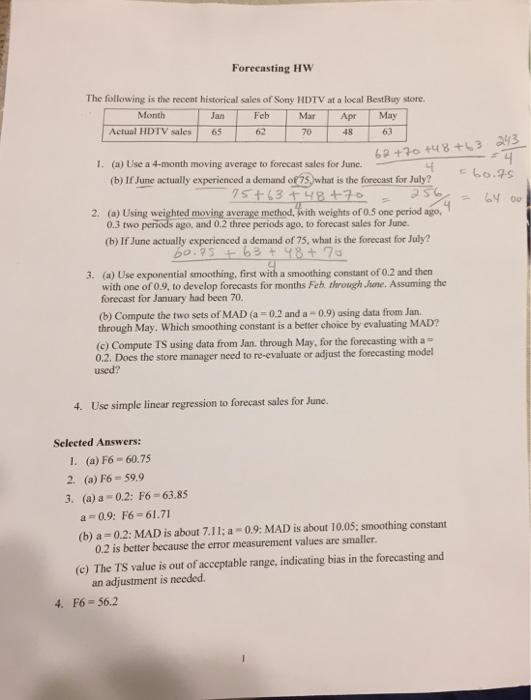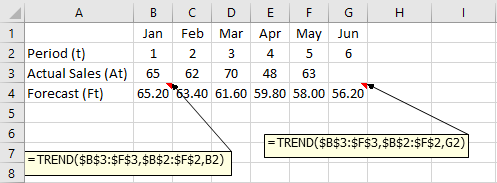# Question & Answer: Forecasting HW The following is the recent historical sales of Sony HDTV at a local…..

For following questions I have different answers can you please explain it if you can. I’ll appreciate it.1

(a) F6 = (62+70+48+63) / 4 = 60.75

Don't use plagiarized sources. Get Your Custom Essay on
Question & Answer: Forecasting HW The following is the recent historical sales of Sony HDTV at a local…..
GET AN ESSAY WRITTEN FOR YOU FROM AS LOW AS \$13/PAGE

(b) F7 = (70+48+63+75) / 4 = 64.00

2.

(a) F6 = (0.2*70 + 0.3*48 + 0.5*63) = 59.9

(b) F7 = (0.2*48 + 0.3*63 + 0.5*75) = 66.0

3.

(a)

 Jan Feb Mar Apr May Jun Actual Sales (At) 65 62 70 48 63 Forecast [Ft = Ft-1 + 0.2(At-1 – Ft-1)] 70 69 67.6 68.08 64.06 63.85
 Jan Feb Mar Apr May Jun Actual Sales (At) 65 62 70 48 63 Forecast [Ft = Ft-1 + 0.9(At-1 – Ft-1)] 70 65.5 62.35 69.24 50.12 61.71

(b)

 Jan Feb Mar Apr May MAD Actual Sales (At) 65 62 70 48 63 Forecast [Ft = Ft-1 + 0.2(At-1 – Ft-1)] 70 69 67.6 68.08 64.06 Absolute Deviation |At – Ft| 5 7 2.4 20.08 1.064 7.1088 Forecast [Ft = Ft-1 + 0.9(At-1 – Ft-1)] 70 65.5 62.35 69.24 50.12 Absolute Deviation |At – Ft| 5 3.5 7.65 21.24 12.88 10.0523

Since MAD with alpha = 0.2 is smaller, chose alpha = 0.2

(c)

 Jan Feb Mar Apr May Actual Sales (At) 65 62 70 48 63 Forecast [Ft = Ft-1 + 0.2(At-1 – Ft-1)] 70 69 67.6 68.08 64.06 Absolute Deviation |At – Ft| 5 7 2.4 20.08 1.064 Cumulative MAD 5 6 4.8 8.62 7.109 Error (At – Ft) -5 -7 2.4 -20.08 -1.064 RSFE -5 -12 -9.6 -29.68 -30.74 TS = RSFE / MAD -1.00 -2.00 -2.00 -3.44 -4.32

Note that the TS values are getting outside the limit of [-2.0, 2.0], So, the forecast is biased

4.

Regression using Excel shortcut method

 Jan Feb Mar Apr May Jun Period (t) 1 2 3 4 5 6 Actual Sales (At) 65 62 70 48 63 Forecast (Ft) 65.20 63.40 61.60 59.80 58.00 56.20